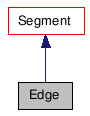Public Member Functions
Edge Class Reference

## Detailed Description

The Edge object gives access to the geometry edges of an X3DObject's primitive.

Edges are the PolygonMesh-specifics of Segments. You can reach edges from PolygonFace::GetEdges and PolygonMesh::GetEdges.

CEdgeRefArray, Segment, Vertex, PolygonMesh, Geometry
Example:
```        using namespace XSI;
Application app;
Model root = app.GetActiveSceneRoot();

X3DObject myCube;
root.AddGeometry( L"Cube", L"MeshSurface", L"", myCube );

PolygonMesh mesh( myCube.GetActivePrimitive().GetGeometry() );

Edge edge( mesh.GetEdges().GetItem(0) );
app.LogMessage( L"This object is an: " + edge.GetClassIDName() );
```

`#include <xsi_edge.h>`

Inheritance diagram for Edge:[legend]

List of all members.

## Public Member Functions

Edge ()
~Edge ()
Edge (const CRef &in_ref)
Edge (const Edge &in_obj)
bool  IsA (siClassID in_ClassID) const
siClassID  GetClassID () const
Edge operator= (const Edge &in_obj)
Edge operator= (const CRef &in_ref)
CVertexRefArray  GetVertices () const
CPolygonNodeRefArray  GetNodes () const
CVertexRefArray  GetNeighborVertices (LONG in_lDistance=1) const
CEdgeRefArray  GetNeighborEdges (LONG in_lDistance=1) const
CPolygonFaceRefArray  GetNeighborPolygons (LONG in_lDistance=1) const
CEdgeRefArray  GrowNeighborEdges (LONG in_lDistance=1) const
double  GetCrease () const
bool  GetIsHard () const
bool  GetIsBoundary () const

## Constructor & Destructor Documentation

 Edge ( )

Default constructor.

 ~Edge ( )

Default destructor.

 Edge ( const CRef & in_ref )

Constructor.

Parameters:
 in_ref constant reference object.
 Edge ( const Edge & in_obj )

Copy constructor.

Parameters:
 in_obj constant class object.

## Member Function Documentation

 bool IsA ( siClassID in_ClassID ) const `[virtual]`

Returns true if a given class type is compatible with this API class.

Parameters:
 in_ClassID class type.
Returns:
true if the class is compatible, false otherwise.

Reimplemented from Segment.

 siClassID GetClassID ( ) const `[virtual]`

Returns the type of the API class.

Returns:
The class type.

Reimplemented from Segment.

 Edge& operator= ( const Edge & in_obj )

Creates an object from another object. The newly created object is set to empty if the input object is not compatible.

Parameters:
 in_obj constant class object.
Returns:
The new Edge object.
 Edge& operator= ( const CRef & in_ref )

Creates an object from a reference object. The newly created object is set to empty if the input reference object is not compatible.

Parameters:
 in_ref constant class object.
Returns:
The new Edge object.

Reimplemented from Segment.

 CVertexRefArray GetVertices ( ) const

Returns the vertices at the ends of this edge.

Returns:
An array of Vertex objects
 CPolygonNodeRefArray GetNodes ( ) const

Returns the PolygonNode objects adjacent to this edge.

Returns:
An array of PolygonNode objects
 CVertexRefArray GetNeighborVertices ( LONG in_lDistance = `1` ) const

Returns an array of Vertex neighbors within a given distance.If the distance is 1, the 2 Vertex objects comprising the current Edge are returned.

Parameters:
 in_lDistance Value Represents the degree of neighborhood (eg., degree=2 for an edge means its adjacent vertices plus the adjacent vertices of the adjacent vertices).
Returns:
An array of Vertex objects
 CEdgeRefArray GetNeighborEdges ( LONG in_lDistance = `1` ) const

Returns an array of Edge neighbors within a given distance.If the distance is 1 the Edge objects are returned in counter-clockwise order.

Parameters:
 in_lDistance Value Represents the degree of neighborhood (eg., degree=2 for an edge means its adjacent vertices plus the adjacent vertices of the adjacent vertices).
Returns:
An array of Edge objects
 CPolygonFaceRefArray GetNeighborPolygons ( LONG in_lDistance = `1` ) const

Returns an array of PolygonFace neighbors within a given distance.If the distance is 1 the adjacent PolygonFace objects are returned (1 if border edge, 2 otherwise).

Parameters:
 in_lDistance Value representing the degree of neighborhood (eg., degree=2 for an edge means its adjacent vertices plus the adjacent vertices of the adjacent vertices).
Returns:
An array of PolygonFace objects
 CEdgeRefArray GrowNeighborEdges ( LONG in_lDistance = `1` ) const

Returns an array of Edge objects that are adjacent to this Edge object, within a given distance.

Parameters:
 in_lDistance Value representing the degree of neighborhood (eg., degree=2 for an edge means its adjacent vertices plus the adjacent vertices of the adjacent vertices).
Returns:
An array of Edge objects
 double GetCrease ( ) const

Returns the crease value associated with this edge.

Returns:
The crease value between 0.0 and 10.0.
Since:
3.5.1
 bool GetIsHard ( ) const

Returns whether the edge is hard or not.

Returns:
True if the edge is hard, false if not.
Since:
3.5.1
 bool GetIsBoundary ( ) const

Returns whether the edge is a boundary or not.

Returns:
True if the edge is a boundary, false if not.
Since:
4.2

The documentation for this class was generated from the following file: